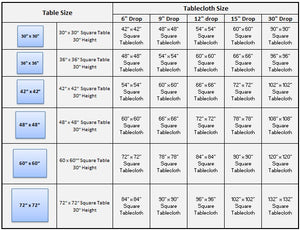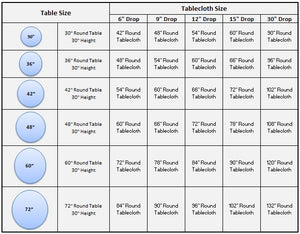# News — Tablecloth Sizing Guide

## Rectangle Tablecloth Sizing Guide

Posted by WoW Imprints onHow to Calculate Tablecloth Size for Rectangle Tables? Step 1: Measure your Table Width and Length.Step 2: Decide the Tablecloth drop you need.Tablecloth Size = (Table Width + 2 times (drop)) x (Table Width + 2 times ( drop))Example:If your Table Width is  30 inch and Length is 72 inch and you need 30" drop to the floor.Rectangle Tablecloth Size = (30 inch + 2 times (30")) + (72 inch + 2 times (30")) Rectangle Tablecloth Size = (30"+60") x (72"+60")Rectangle Tablecloth Size = 90" x 132"You will need  to order 90 x 132 inch Rectangle Tablecloth for your 30 x...

## Square Tablecloth Sizing Guide

Posted by WoW Imprints onHow to Calculate Tablecloth size for Square Tables? Step 1: Measure your Table Width and Length.Step 2: Decide the Tablecloth drop you need.Tablecloth Size = (Table Width + 2 times (drop)) x (Table Width + 2 times ( drop)) Example:If your Table Width is  60 inch and Length is 60 inch and you need 15" drop to the floor.Tablecloth Size = (60 inch + 2 times (15")) + (60 inch + 2 times (15"))Tablecloth Size = (60"+30") x (60"+30")Tablecloth Size = 90"x90"You will need  to order 90 x 90 inch Square Tablecloth for your 60 x 60 inch Square Table.What is the ideal...

## Round Tablecloth Sizing Guide

Posted by WoW Imprints onHow to Calculate Tablecloth Size for Round Tables? Step 1: Measure your Round Table Diameter.Step 2: Decide the Tablecloth drop you need.Tablecloth Size = Table Diameter + 2 times (drop) Example:If your Round Table Diameter is 60 inch and you need 15 inch drop to the floor.Round Tablecloth Size = 60 inch + 2 times (15" drop) Round Tablecloth Size = 60 "+ 30"Round Tablecloth Size = 90"You will need to order 90 inch Round Tablecloth for your 60 inch Round Table. What is the ideal tablecloth drop?  How many inches should a tablecloth hang over? Click to read. Round Tablecloth Sizing Chart ...